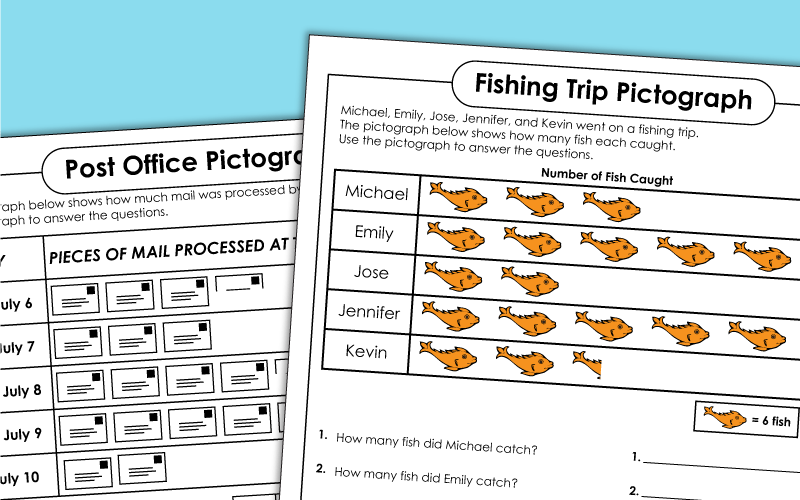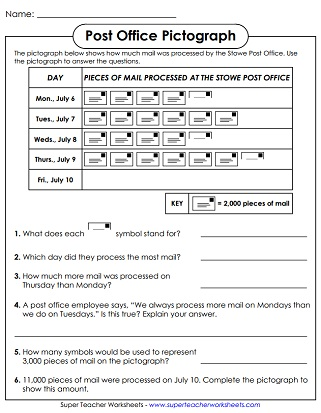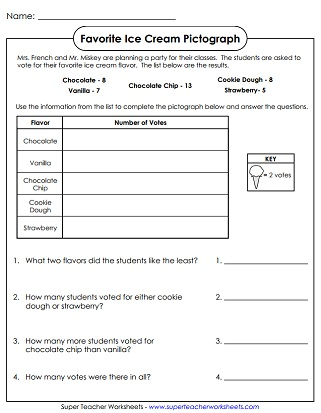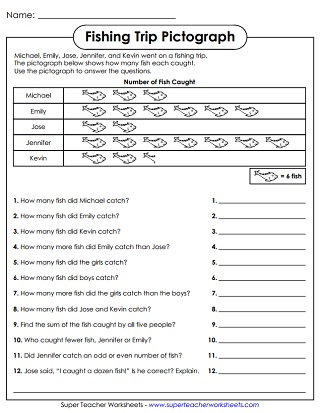# Pictograph Worksheets

A pictograph, or picture graph, is a graph that uses symbols to represent data.  Students will read and interpret data, as well as make graphs of their own.## Basic Pictographs

Students complete the simple pictograph about favorite ice cream flavors; Includes half symbols; Approximately 2nd-3rd grade level
Use the information to complete the pictograph, then answer the questions that follow; No half symbols; Approximately 2nd grade level
Complete the popcorn sale pictograph and answer the questions; No half symbols; Approximately 2nd-3rd grade level
Simple graph shows lemonade stand profits.  Scale counts by 5s and does NOT include half symbols.
Basic pictograph has bear shapes to represent stuffed animals.  Students will determine how many stuffed animal each person has.  Does NOT include half symbols.

## Intermediate Pictographs

Students analyze the data on the chart to answer questions.  Scale counts by 2,000s and does include half symbols.
How many customers visit French's each year.  To find out, students examine the pictograph.  Requires counting by thousands and includes half symbols.
Students complete the pictograph using data in a table.  Then, they complete the short answer questions that follow.  Pictograph counts by 10s and does include half symbols.
Students are given a pictograph with data for a cupcake bakery. They use the data in the graph to answer the questions.
This picture graph features bird symbols that count by twos.  Students must use the graph to answer questions.  Requires adding, subtracting, and inferencing.  Includes half symbols.

On this pictograph, students count by 6s to determine how many fish were caught.  Includes half symbols.
Count by 4s to determine how many pumpkins each person carved and answer questions.  Many questions involve higher-level thinking.  Includes half symbols.

## Any Level

Make your own pictograph with this blank template.
Bar Graph Worksheets

Printable worksheets with bar graphs and questions

Line Graph Worksheets

Line graph worksheets show a change in data over a period of time

## Images of Our WorksheetsMy Account
Site Information